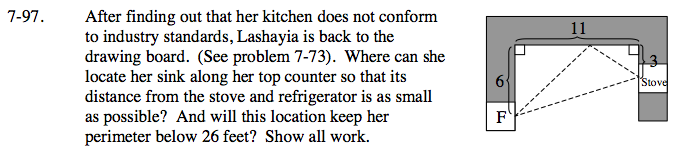### Home > GC > Chapter 7 > Lesson 7.2.6 > Problem7-97

7-97.This problem is similar to the Interior Design problem from Lesson 7.1.3. How did you find the solution for that problem?

For the shortest possible distance, the two right triangles should be similar.

Set up equivalent ratios of corresponding sides: Remember the side that is length 11, can be split into two lengths x and 11 − x.

$\frac{6}{x}= \frac{3}{11-x}$

Solve for x.